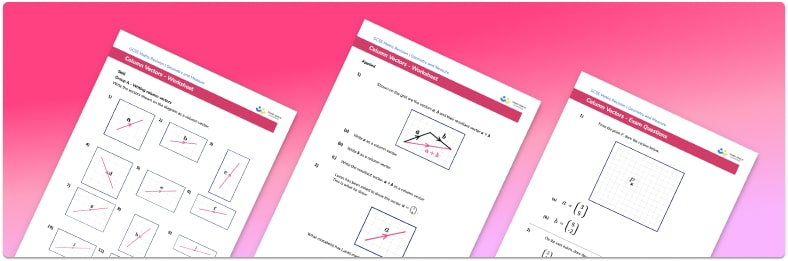# Column Vectors Worksheet• Section 1 of the column vectors worksheet contains 20+ skills-based column vectors questions, in 3 groups to support differentiation
• Section 2 contains 4 applied column vectors questions with a mix of worded problems and deeper problem solving questions
• Section 3 contains 4 foundation and higher level GCSE exam style column vectors questions
• Answers and a mark scheme for all column vectors questions are provided
• Questions follow variation theory with plenty of opportunities for students to work independently at their own level
• All questions created by fully qualified expert secondary maths teachers
• Suitable for GCSE maths revision for AQA, OCR and Edexcel exam boards

• This field is for validation purposes and should be left unchanged.

You can unsubscribe at any time (each email we send will contain an easy way to unsubscribe). To find out more about how we use your data, see our privacy policy.

### Column vectors at a glance

Column vectors describe a movement. The top number of a column vector is the x component – it tells us where to move in the x direction (right and left). The bottom number is the y component – it tells us where to move in the y direction (up and down). For example, the column vector of 3 on top of  negative 4 in large round brackets describes a movement of 3 in the positive x direction (right) and 4 in the negative y direction (down).

A column vector can be multiplied by a scalar. When multiplying a vector by a scalar, we multiply both components of the vector by the scalar. Two vectors are parallel if one is a scalar multiple of the other. Multiplying a vector by -1 would give a vector of the same length but in the opposite direction.

We can perform vector addition and subtraction with column vectors. To do this, we add or subtract the x components of each vector and then add or subtract the y components.

The magnitude of a vector is its length. The magnitude of a column vector can be found using Pythagoras’ Theorem. By drawing lines representing the x component and y component of the vector, we can construct a right angle triangle. The magnitude is the length of the hypotenuse of the triangle formed.

Looking forward, students can then progress to additional geometry worksheets, for example the vector problems worksheet, the transformations worksheet, or the Pythagoras’ Theorem worksheet.For more teaching and learning support on Geometry our GCSE maths lessons provide step by step support for all GCSE maths concepts.

## Do you have KS4 students who need more focused attention to succeed at GCSE?There will be students in your class who require individual attention to help them succeed in their maths GCSEs. In a class of 30, it’s not always easy to provide.

Help your students feel confident with exam-style questions and the strategies they’ll need to answer them correctly with our dedicated GCSE maths revision programme.

Lessons are selected to provide support where each student needs it most, and specially-trained GCSE maths tutors adapt the pitch and pace of each lesson. This ensures a personalised revision programme that raises grades and boosts confidence.

Find out more